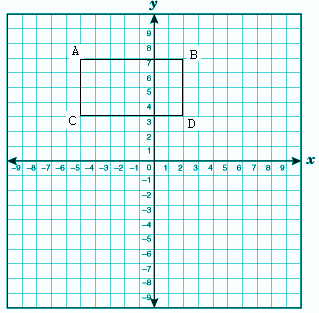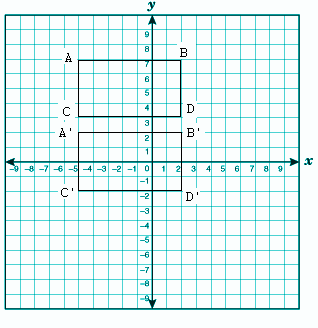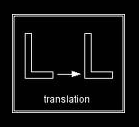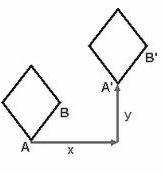# Translation

In Geometry, the four basic translation or transformations are:

• Translation
• Reflection
• Rotation
• Dilation or Resizing

In this article, let’s discuss the meaning of “Translation” in Maths, translation in the coordinate plane and examples in detail.

## Translation Definition

Translation means the displacement of a figure or a shape from one place to another. In translation, a figure can move upward, downward, right, left or anywhere in the coordinate system. In translation, only the position of the object changes, its size remains the same.

It can change their path like at first it moves left then after it will take a turn right then again right and so on. If one point of a shape such as a triangle, rectangle, square, line, circle and so on., move five units in forwarding direction, then all the points will move by five units in the same direction. Suppose one point of a triangle moves four units in left direction then all points of triangle move four units in the left direction

## Translation in Coordinate Plane

In the below problem, let us discuss how the translation happens in the coordinate plane.

A rectangular graph with the points A(-5,7), B(2,7), C(-5,3), D(2,3) as shown in the figure. Shift it by five units downward. Find the translated figure?Solution:

If a figure is translated by five units in a downward direction, then there is no change in x- coordinate or horizontal direction

So, we have to subtract by -5 in the y coordinate

Therefore, we get A(-5, 7)

After translation coordinate will be (x-0, y-5)

= (-5-0, 7-5)

Hence, (-5, 2) is a translated coordinate.

For B(2, 7), the translated coordinate will be (x-0, y-5)

= (2-0, 7-5)

Hence, (2, 2) is a translated coordinate.

For C(-5, 3), the translated coordinate will be (x-0, y-5)

= (-5-0, 3-5)

Hence, (-5, -2) is a translated coordinate.

For D(2, 3), the translated coordinate will be (x-0, y-5)

= (2-0, 3-5)

Hence, (2, -2) is a translated coordinate.

Therefore, the translated figure for the given coordinate is :### Translation Example

Some examples of translation are given below:

Example 1:

An example of translation towards the right is given below.Here The figure “L” is shifted in the right direction with a fixed distance. The shape and size of the image is the same after the translation on the position of image changes

Example 2:

Here is an example of translating images in more than one direction.Here the rhombus is first moved x unit towards the right direction and then moved y unit in an upward direction. Thus, the rhombus AB is shifted to a new position A’B’.

Stay tuned with BYJU’S – The Learning App and also download the app to explore more Maths-related articles.

 Related Links Transformations Reflection Rotation Dilation